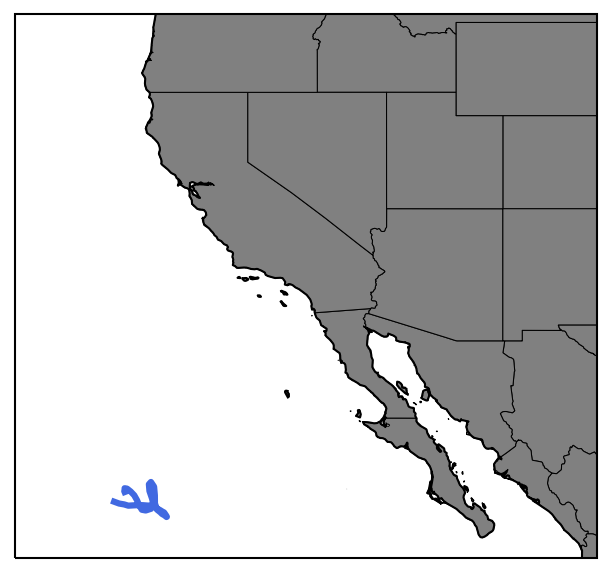# Bonus Activity 4 - Loading and Plotting Argo Float Data¶

2020 Data Labs REU

Written by Sage Lichtenwalner, Rutgers University, June 9, 2020

In this notebook we will cover some of basics of loading and plotting ARGO drifter in python.

Becuase this dataset inclues a depth dimension, this allows us to demonstrate several addtional graph types you will commonly see in oceanography, including profiles and TS diagrams.

In :
# Notebook setup
import xarray as xr
!pip install netcdf4

import matplotlib.pyplot as plt

Requirement already satisfied: netcdf4 in /usr/local/lib/python3.6/dist-packages (1.5.3)
Requirement already satisfied: cftime in /usr/local/lib/python3.6/dist-packages (from netcdf4) (1.1.3)
Requirement already satisfied: numpy>=1.7 in /usr/local/lib/python3.6/dist-packages (from netcdf4) (1.18.5)


# ARGO Drifter Data¶

While timeseries datasets at fixed points are very useful, the ocean is a deep place with a lot of interesting features and processes that vary with depth.

Thus, it's also important to be able to visualize data in ways that emphasize the depth dimension, just as we've emphasized the time dimension above.

To do so, we're going to load data from the Global Argo Float program, which had 3,962 active drifters around the world as of 6/6/2020.Unfortunately, because of the complexity and international nature of the program, there isn't one "perfect" source to retrieve Argo data, or even to search for drifters you may be interested in. However, I found the following sites helpful.

For the purposes of this activity, I picked an active drifter at random. Let's see what #5906017 can tell us about the ocean.

In :
# Let's download a datafile to our serve (see the files tab)

--2020-06-09 18:42:59--  https://data.nodc.noaa.gov/argo/gadr/data/aoml/5906017/nodc_5906017_prof.nc
Resolving data.nodc.noaa.gov (data.nodc.noaa.gov)... 140.90.235.40
Connecting to data.nodc.noaa.gov (data.nodc.noaa.gov)|140.90.235.40|:443... connected.
HTTP request sent, awaiting response... 200 OK
Length: 1167788 (1.1M) [application/x-netcdf]
Saving to: ‘nodc_5906017_prof.nc.2’

nodc_5906017_prof.n 100%[===================>]   1.11M   648KB/s    in 1.8s

2020-06-09 18:43:02 (648 KB/s) - ‘nodc_5906017_prof.nc.2’ saved [1167788/1167788]


In :
# Load the dataset using xarray
data = xr.open_dataset('nodc_5906017_prof.nc')
data


As you can see, this dataset is a bit more complicated than our mooring timeseries dataset.

Argo uses the dimensions n_levels (for depth) and n_prof (for time).

In :
# Quick Timeseries Profile plot of Temperature
plt.gca().invert_yaxis()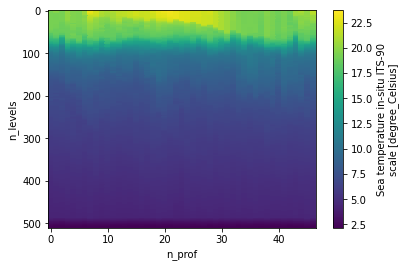Note, when possible, you should use the _adjusted variables, as those have been corrected. However, if you are interested in more recent data that hasn't been corrected yet, you will need to use the regular variable names (e.g. temp instead of temp_adjusted).

## Profile Plot¶

Now that we have the dataset loaded, let's create a profile plot of a single profile.

In :
# Profile Plot
nprof = 25 #Specify a profile to plot

plt.xlabel('Temperature (C)')
plt.ylabel('Pressure (dbar)')
plt.title('Argo Profile from %s' % data.juld[nprof].dt.strftime('%a, %b %d %H:%M').values)

plt.gca().invert_yaxis() #Flip the y-axis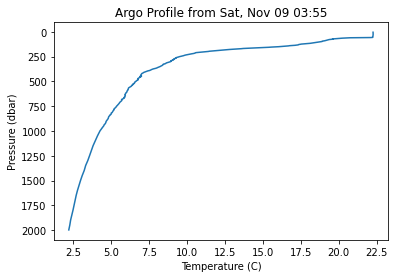Now let's get a little fancier and plot profiles of 2 variables.

In :
# Profile Plot
# Subplot example
fig, (ax1,ax2) = plt.subplots(1,2, sharey=True, figsize=(10,6))

nprof = 0 # Fist profile

nprof = 25 # Middle-ish profile

nprof = -1 # Last profile

ax1.set_ylabel('Pressure (dbar)')
ax1.set_xlabel('Temperature (C)')
ax2.set_xlabel('Salinity')
ax1.invert_yaxis()
ax1.legend()

# Add some gridlines
ax1.grid()
ax2.grid()

# Add a super title
fig.suptitle('Argo Float #%d' % data.platform_number[nprof].values, fontweight='bold', fontsize=16);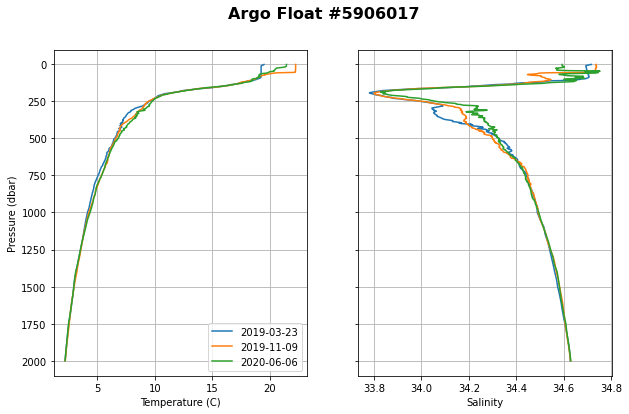### Activity Break¶

Repeat the steps above to create profile plot of temperature and salinity using Float 5905077.

Note that this float has a lot of other variables that could be investigated.

In :
# Your Turn - Download the data

In :
# Your Turn - Load the data

In :
# Your Turn - Profile plot


## T-S Diagram¶

Another popular plot in oceanography is the T-S Diagram, or Temperature-Salinity plot. It is commonly used to diagnose water masses in the global ocean, and to compare density stability of a water column profile (especially when lines of constant density are also included).

Basically, it's just a scatterplot of temp and salinity.

In :
# TS Diagram
nprof = 25 #Selected profile
plt.xlabel('Salinity')
plt.ylabel('Temperature (°C)')
plt.grid()

plt.title('Argo Float #%d' % data.platform_number[nprof].values, fontweight='bold');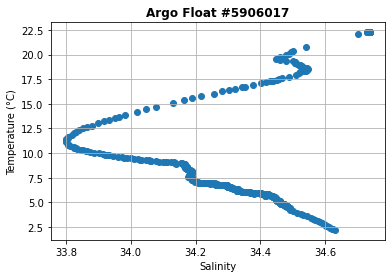That's nice, but we can also use a colored scatterplot to show the depth dimension.

In :
# T-S Diagram with depth
plt.figure(figsize=(8,6))

nprof = 25 #Selected profile
plt.xlabel('Salinity');
plt.ylabel('Temperature (°C)')

cbh = plt.colorbar();
cbh.set_label('Pressure (dbar)')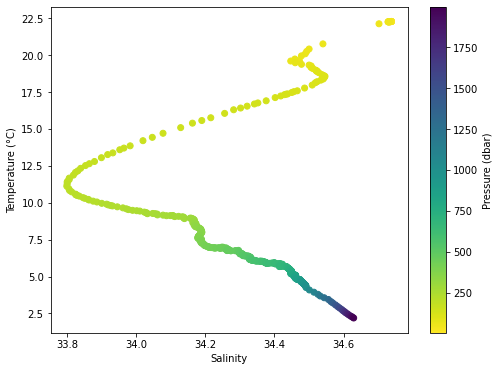We used the default python colorbar for this plot (viridis), but there is a much larger colormap collection available.

With a little bit more code, we can add lines of constant density. This was adapted from the Ocean Python T-S Diagram example, but we will use meshgrid instead, since it makes the code a bit simpler.

To calculate density, we will need the wonderful seawater library.

In :
!pip install seawater
import seawater
import numpy as np

Requirement already satisfied: seawater in /usr/local/lib/python3.6/dist-packages (3.3.4)
Requirement already satisfied: numpy in /usr/local/lib/python3.6/dist-packages (from seawater) (1.18.5)

In :
# TS Diagram with density contours
plt.figure(figsize=(8,6))

# Calculate the density lines
x = np.arange(33, 35, .1)
y = np.arange(2, 23, .5)
X, Y = np.meshgrid(x, y)
Z = seawater.eos80.dens0(X,Y) - 1000 # Substract 1000 to convert to sigma-t

# Plot the contour lines
CS = plt.contour(X, Y, Z, colors='grey', linestyles='dashed', levels=np.arange(22,30,.5))
plt.clabel(CS, inline=1, fontsize=10, fmt='%0.1f')

# Plot the data
nprof = 25 #Selected profile
plt.xlabel('Salinity');
plt.ylabel('Temperature (°C)')
plt.title('Argo Float #%d on %s' % (data.platform_number[nprof].values, data.juld[nprof].dt.strftime('%Y-%m-%d').values), fontweight='bold');

# Add a colorbar
cbh = plt.colorbar(label='Pressure (dbar)');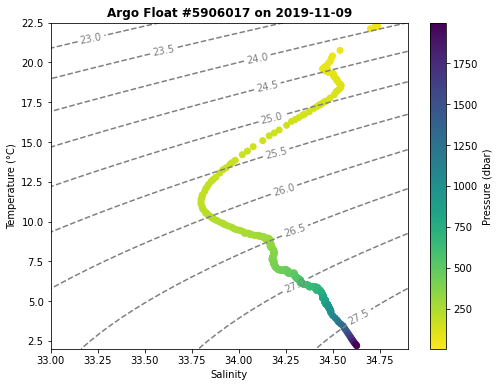## Float Track Map¶

In :
# Simple map of a float track
plt.figure(figsize=(8,6))
plt.plot(data.longitude, data.latitude, c='lightgrey')
plt.scatter(data.longitude, data.latitude, c=data.juld, cmap='RdYlBu')

# Crude profile labels
for jj in [1,25,-1]:
plt.text(data.longitude[jj]+.02, data.latitude[jj]+.02, data.n_prof[jj].values)

# Add a colorbar
cbar = plt.colorbar();

# Fix the colorbar ticks
import pandas as pd # We need pandas for this
cbar.ax.set_yticklabels(pd.to_datetime(cbar.get_ticks()).strftime(date_format='%Y-%m-%d'));

# Set the aspect ratio to pseudo-Mercator
plt.gca().set_aspect(1 / np.cos(np.deg2rad( np.mean(plt.ylim()) )))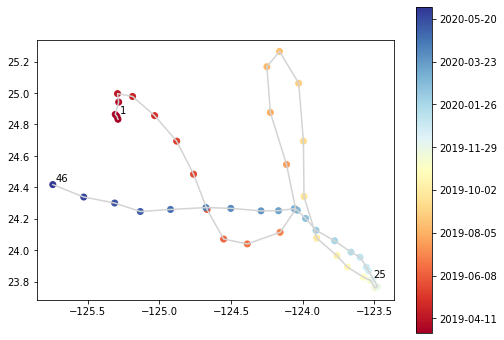## Fancier Maps with Cartopy¶

The cartopy library provides a lot of great features to create your own custom maps, though it takes a lot of patience to get it to work correctly.

In :
# Install Cartopy in Google Colab
!apt-get -V -y -qq install python-cartopy python3-cartopy
!pip uninstall shapely -y
!pip install shapely --no-binary shapely

Uninstalling Shapely-1.7.0:
Successfully uninstalled Shapely-1.7.0
Collecting shapely
Skipping wheel build for shapely, due to binaries being disabled for it.
ERROR: albumentations 0.1.12 has requirement imgaug<0.2.7,>=0.2.5, but you'll have imgaug 0.2.9 which is incompatible.
Installing collected packages: shapely
Running setup.py install for shapely ... done
Successfully installed shapely-1.7.0

In :
import cartopy.crs as ccrs
import matplotlib.pyplot as plt
from cartopy.feature import NaturalEarthFeature

In :
fig = plt.figure(figsize=(6.4,4.8),dpi=150)

ax = plt.axes(projection=ccrs.PlateCarree())
ax.set_extent([-130, -105, 22, 45])

# Basemaps
states = NaturalEarthFeature(category="cultural", scale="10m",
facecolor="none",